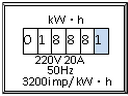（1）测量原理是________。（用记录的字母、电能表上的技术参数表达）

（2）断开家中其他用电器，只闭合电水壶开关，观察并记录时间t内________。

（3）所测量的电热水壶功率表达式为________。

（4）若发现测量的功率比铭牌上标注的额定功率略小一些，可能原因是以下的哪几个________（填序号）

①实际电压偏低；②电热丝阻值偏大；③电热丝阻值偏小；④烧水过程有热损耗。$$P=\frac{W}{t}=\frac{\frac{\mathrm{n}}{3200} \mathrm{~kW} \cdot \mathrm{h}}{t}=\frac{\mathrm{n}}{3200 \mathrm{t}} \mathrm{kW \cdot h} \text {; }$$

(2) (1) 由 $\mathrm{P}=\frac{\mathrm{U}^2}{\mathrm{R}}$ 可知, 实际电压偏低时, 会造成测量的功率比铭牌上标注的额定功率略小;
(2) 由 $\mathrm{P}=\frac{\mathrm{U}^2}{\mathrm{R}}$ 可知, 电热丝阻值偏大时, 会造成测量的功率比铭牌上标注的额定功率略小;
(3) 由 $\mathrm{P}=\frac{\mathrm{U}^2}{\mathrm{R}}$ 可知, 电热丝阻值偏小时, 会造成测量的功率比铭牌上标注的额定功率略大;
(4) 由 $P=\frac{\mathbb{W}}{\mathrm{t}}=\frac{\mathrm{Q}}{\mathrm{t}}$ 可知, 烧水过程有热损耗, 不会影响消耗电能的测量以及通电时间的测量, 即不会影 响测量结果。

(1) $\mathrm{P}=\frac{\mathrm{W}}{\mathrm{t}}$
（2）电能表指示灯闪炼的次数 $n$;
(3) $\mathrm{P}=\frac{\mathrm{n}}{3200 \mathrm{t}} \mathrm{kW} \cdot \mathrm{h}$ ；
(4)①②